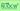# Pre-college Mathematics *Optional subjectLecturer Yukio ISHIDA, Professor G30, 2015 Fall G30 (2・1.5 hours / session One session / week 15 weeks / semester)

### Course Overview

This course is a companion course to Calculus I. It aims to help students with little or no precalculus knowledge to master the basic calculus material in preparation for the more advanced course of Calculus I. This is done by reviewing high school mathematics.

### Course Prerequisites

There are no particular prerequisites for this course. Basic algebraic computation capabilities will ease the learning.

CONTENTS

### Notice for Students

This course is an optional subject which does not count towards the number of credits required for graduation in any program at Nagoya University. This course will be more or less demanding depending on the initial level in mathematics. In case of difficulties, it is recommended to study in e-learning lecture introduced in the first lesson.

### Textbook

Handouts are delivered.

### Reference Book

E-learning in the Nagoya University website (This is introduced in the first lecture).

### Lecture Videos

Session Lectures Video Notes
Course I Functions and Equations
1 Polynomials and Factoring Video
(19:45)
Note
2 Algebraic Equations and Functions Video
(18:35)
Note
3 Linear and Quadratic Inequalities Video
(16:08)
Note
4 Trigonometric Functions Video(I) Video
(14:09)
Note
5 Trigonometric Functions Video(II) Video
(17:25)
Note
6 Trigonometric Functions Video(III) Video
(15:01)
Note
7 Complex Numbers Video
(23:10)
Note
8 Exponential Functions Video
(22:42)
Note
9 Fractional Functions and Irrational Functions Video
(15:33)
Note
10 Inverse Functions Video
(14:42)
Note
11 Common Logarithms Video
(30:14)
Note
12 Law of Sines and Law of Cosines Video
(16:41)
Note
13 Applications of Trigonometric Functions Video
(14:25)
Note
14 Graphs and Equations Video(I) Video
(22:20)
Note
15 Graphs and Equations Video(II) Video
(25:10)
Note
Course II Calculus
1 Limit of Functions and Derivatives Video
(26:28)
Note
2 Derivative and Graphs Video
(22:07)
Note
3 Differentiation Formulas Video
(16:55)
Note
4 Derivatives of Trigonometric Functions Video
(18:01)
Note
5 Derivatives of Logarithmic Functions and Exponential Functions Video
(19:33)
Note
6 Applications of Derivatives to Equations and Inequality Video
(19:54)
Note
7 Application to Physics Video
(22:52)
Note
8 Approximation of a Function Video
(20:04)
Note
9 Antiderivatives Video
(30:03)
Note
10 Definite Integrals Video
(20:58)
Note
11 Estimating Area by Rectangles Video
(14:41)
Note
12 Application of Integrals Video(1) Video
(22:24)
Note
13 Application of Integrals Video(2) Video
(27:03)
Note
14 Differential Equations Video(1) Video
(21:20)
Note
15 Differential Equations Video(2) Video
(19:07)
Note
Course III Linear Algebra
1 Basic Rules of Vectors Video
(23:58)
Note
2 Inner Product Video
(22:06)
Note
3 Vector Equations Video
(15:16)
Note
4 Basic Rules of Matrices Video
(16:09)
Note
5 Multiplication of Matrices Video
(16:53)
Note
6 Inverse Matrix and Simultaneous Equations Video
(18:08)
Note
7 Linear Transformation Video
(14:20)
Note